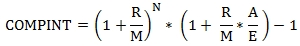# SQL Server accrued compound interest function

COMPINT

Updated: 30 November 2013

Use the scalar function COMPINT to calculate the accrued interest for a security where interest is compounded periodically and paid at maturity.Where:
R = the coupon interest rate as a decimal
M = the number of compounding periods per year
N = the number of whole coupons prior to the settlement date
A = the number of accrued days in the coupon period in which the settlement occurs
E = the number of days as specified by the basis code for the coupon period in which the settlement occurs.
Syntax
SELECT [wctFinancial].[wct].[COMPINT](
<@Basis, nvarchar(4000),>
,<@Rate, float,>
,<@IssueDate, datetime,>
,<@Settlement, datetime,>
,<@Maturity, datetime,>
,<@CompFreq, int,>)
Arguments
@Basis
is the type of day count to use. @Basis is an expression of the character string data type category.
 Basis Day count basis 0 US (NASD) 30/360 1 Actual/Actual 2 Actual/360 3 Actual/365 4 European 30/360
@Rate
the coupon rate of the security expressed in decimal terms. @Rate is an expression of type float or of a type that can be implicitly converted to float.
@IssueDate
the issue date of the security; the first interest accrual date. @IssueDate is an expression of a datetime data type or of a type that can be implicitly converted to datetime.
@Settlement
the settlement date occurring within a coupon period of the security; interest is accrued from @IssueDate through to @Settlement. @Settlement is an expression of a datetime data type or of a type that can be implicitly converted to datetime.
@Maturity
the maturity date of the bond. @Maturity is used to determine the coupon dates. @Maturity is an expression of a datetime data type or of a type that can be implicitly converted to datetime.
@CompFreq
the number of times the coupon is compounded annually. For annual compounding, @CompFreq = 1; for semi-annual, @CompFreq = 2; for quarterly, @CompFreq = 4, and for monthly, @CompFreq = 12. @CompFreq is an expression of type float or of a type that can be implicitly converted to float.
Return Type
float
Remarks
·         If @CompFreq not 1, 2, 4, or 12 an error will be returned.
·         @Issuedate <= @Settlement <= @Maturity.
·         For bonds with an odd first or an odd last coupon period (or both), use ODDCOMPINT.
·         COMPINT returns a factor. To calculate the monetary value of the accrued interest, you should multiply this factor by the face amount of the bond.
Examples
A 3-year bond, compounding monthly, with settlement in the first coupon period. Interest is accrued on an actual/actual basis.
SELECT wct.COMPINT(
1                --@Basis
,.0175            --@Rate
,'2013-11-15'     --@IssueDate
,'2013-11-29'     --@Settelemt
,'2016-11-15'     --@Maturity
,12               --@CompFreq
) as COMPINT

This produces the following result.
COMPINT
----------------------
0.000680555555555573

The same bond, settling 1 year later.
SELECT wct.COMPINT(
1                --@Basis
,.0175            --@Rate
,'2013-11-15'     --@IssueDate
,'2014-11-29'     --@Settelemt
,'2016-11-15'     --@Maturity
,12               --@CompFreq
) as COMPINT

This produces the following result.
COMPINT
----------------------
0.0183336104248544

By entering the maturity date as the settlement date, we can calculate the total amount of the compound interest at maturity.
SELECT wct.COMPINT(
1                --@Basis
,.0175            --@Rate
,'2013-11-15'     --@IssueDate
,'2016-11-15'     --@Settelemt
,'2016-11-15'     --@Maturity
,12               --@CompFreq
) as COMPINT

This produces the following result.
COMPINT
----------------------
0.0538622573229668

### SupportCopyright 2008-2023 Westclintech LLC         Privacy Policy        Terms of Service